Next: Runaway Discharge in a
Previous: Modeling Red Sprites

# Chapter 4: Spectrum of Red Sprites

We proceed next to model the spectrum of red sprites due to the electron energization by the electric field from lightning. It capitalizes on the fact that there are two distinct timescales. A fast timescale on which a steady state electron distribution function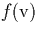is established by balancing the electron energization rate with inelastic loses (see Appendix B), and a slow radiation timescale dominated by interlevel transfer and collisional quenching.

The electron energization is computed with the help of the Fokker-Planck code (described in Appendix B). We assume first that sprites located at a certain height z, and for the sake of definiteness assume that z=80 km [Lyons , 1994; Sentman et al., 1995; Bossipio et al., 1995; Winckler et al., 1996]. Then we discuss a more realistic model of a spatially integrated spectrum of red sprites.

Radiative deexcitation of the excited molecules produces optical flashes that superficially resemble those observed during auroras. However, unlike auroras which last for hours and in which even forbidden transitions need to be considered, red sprites have millisecond duration, so that only N2 transitions faster than a millisecond excited by direct electron impact or through cascades need to be retained (Fig. C.1). The radiative deexcitation involves:

• Direct pumping of the v vibrational level of the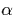electronic state by the electron impact.
• Collisional quenching.

The usage of the stationary equations for the population of vibrational level is justified by the fact that radiative lifetime of the relevant electronic states have to be shorter than the duration T of electromagnetic pulse from lightning in order to be effectively pumped. Therefore, a stationary distribution of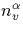is established during the pulse.

Note that since we consider the shape of sprite spectrum, only relative line intensities are of interest. Furthermore, the vibrational-electronic population depends linearly on the electron density (see Eq. (C.2)); as a result the spectrum is not affected by the possible increase in the electron density due to the ionization of the neutral gas by ''hot'' electrons. Using the above computational scheme we find the synthetic source spectrum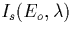of N2 localized at 80 km which includes the first and second positive and first negative bands. It is presented in Fig. 4.1 for two values of the electric field amplitude E0= 35 and 70 V/m. Notice that only the 1P and 2P bands give a distinctive contribution to the source spectrum, while the N2+(1N) band plays only a minor role since it can only be excited by ''tail'' electron having energy in excess of 19 eV. In comparison, this N2+(1N) band is among the brightest in auroras since it is caused by high energy electrons.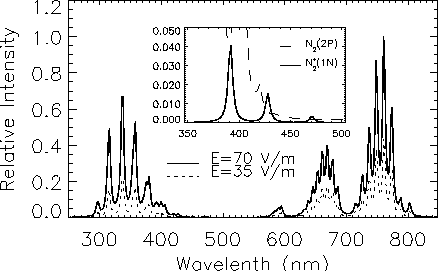The observed spectrum depends on the location of the detector. If observed from space, the spectrum is the same as the source spectrum, while if observed from either ground or airplane it will be distorted by atmospheric attenuation. Atmospheric attenuation depends on the zenith angle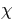of the optical source, the altitude ho of the detector, and on the properties of the atmosphere, such as relative humidity and aerosol density. We consider the following contributions to the attenuation:

• absorption by ozone
• absorption by oxygen
• absorption by water vapor
• Rayleigh scattering by air molecules
• Mie scattering by aerosols.Figure 4.2 reveals the model sprite spectrum as observed by a ground based detector viewing the source at zero zenith angle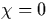. This spectrum differs significantly from the synthetic source spectrum 4.1 . First, the N2(2P) and N2+(1N) bands are attenuated more significantly than the N2(1P) band. This effect is stronger for longer optical paths corresponding to higher zenith angle as seen in Fig 4.3 . By comparing the two peaks of the N2(1P) band, say 5-2 and 4-2 which undergo different absorption, one can estimate the zenith angle of the observed sprite.

From the optical spectrum one can retrieve the intensity of the pumping electric field. This is accomplished by comparing lines either belonging to different bands or to the same band. In the first case the ratio of excitation rates of the corresponding electronic levels depends on the direct pumping of the levels (mainly) and from cascades excitation. In the second case this ratio is controlled by the cascades excitation only.

Since only a few lines belonging to the 1P band have been observed so far [ Mende et al., 1995; Hampton et al., 1996], in what follows we consider the vibrational transitions v - vand v1-v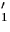belonging to the N2(1P) band. For given values of the relative intensities of two chosen spectral lines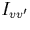and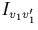we obtain using Eqs. (C.2), (C.3) that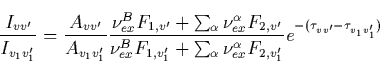(10)
where the summation is over B, C and D electronic states reflecting the effect of excitation and cascade;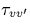andreveal the atmospheric absorption of the corresponding levels.

Generally speaking, one can obtain ratios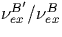,and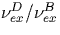by using intensities of three different spectral bands. This allows the evaluation of the electric field amplitude from Fig. C.2. This procedure requires knowledge of the atmospheric attenuation, which includes the zenith angle, as well as the relative humidity, and the aerosol number density. However, if the detector is boarded on a high altitude airplane, the absorption caused by the water vapor and aerosols becomes negligible. Thus the retrieval procedure is simplified. In order to illustrate the opportunities given by the proposed method we consider data from Hampton et al.  presented in Fig. 4.4a which only shows the N2(1P) band. The ratio of the intensities of the 6-3 to the 7-4 transitions is 0.62/0.4. We take into account that for the chosen transitions the largest role is played by the direct pumping of the B level and by the cascade from the Blevel. The difference in the atmospheric attenuations, as we check with our model, was less than a few percent for the zenith angle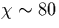. Substituting into Eq. (4.1) the ratio of the intensities we obtain that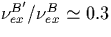, and according to Fig. C.2 this corresponds to the electric field amplitude Eo=35 V/m which at z=80 km is below the breakdown threshold. Note that this estimate was made using noisy data which are not spatially resolved, and can be considered only as an illustrative example. However, the synthetic spectrum calculated for the electric field amplitude E o=35 V/m, the zenith angle of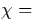80o, and for the detector location of 4.3 km above the sea level, which is shown in Fig. 4.4b, resembles that observed by Hampton et al.  at similar conditions, as revealed by Fig. 4.4a.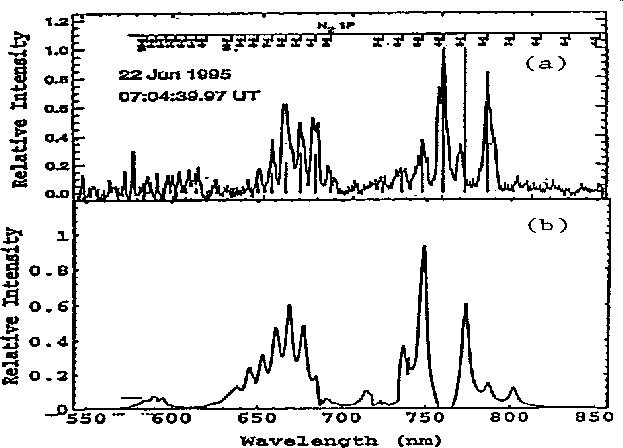To compare with actual spectrum measurements, we must consider the detector column integration, as photons are emitted from different heights under different conditions, e.g. quiver energies, electron densities, neutral densities, collisional quenching, etc. The column integration, Eq. ( C.3), must be carried carefully due to two factors:

• the height dependence of the quiver energy, the electron density, and neutral density;
• the height dependence of the collisional quenching.

We can apply the method we explained above to the electric field produced by our fractal lightning model in the lower ionosphere, as discussed in Chapter 2 and 3. For a definiteness we assume that the lightning discharge has a fractal dimension D=1.2 (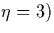with a current peak of Io=100 kA. We chose the field profile at the core of the sprites to carry the spatial integration Eq. (C.3). The result is shown in Fig. 4.5. In fact, for this electric field profile, the relative spectrum does not change considerably from the, properly normalized, spectrum computed for a slab at h=80 km and of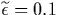eV (35 V/m) revealed by a dashed line, the difference being only 10% for the short wavelengths of the 2P band and less than 1% for the longer wavelengths of the 1P band. We expected that the differences become more pronounce as the sprites spread downward below 70 km.

In conclusion, a model of the red sprite spectrum due to molecular excitation by ionospheric electrons accelerated by the electric field from lightning was developed. The model could allow us to evaluate the electric field amplitude by comparing the intensities of different spectral lines. The model also reveals some differences between the aurora and sprite spectra: in the aurora both permitted and forbidden transitions play a noticeable role, while in sprites only permitted transitions are important. It seems that sprites are produced by electrons of much lesser energy than that of auroral electrons. Unlike the aurora, the collisions between excited molecules could affect sprites only in a few local spots. Sprites are normally observed at high zenith angle, so the spectrum is highly influenced by the atmospheric attenuation. For a given zenith angle and atmospheric constitution (i.e. humidity and aerosol density), the collisional quenching and the atmospheric attenuation can be computed accurately. As a result, if the measurements have good spatial resolution, the model output could in principle yield the spatial profile of the amplitude of the electric field causing the sprite.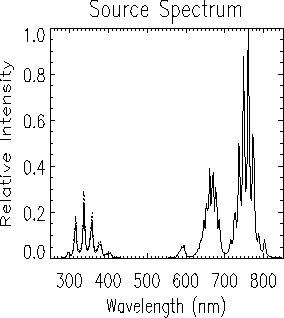Next: Runaway Discharge in a
Previous: Modeling Red Sprites